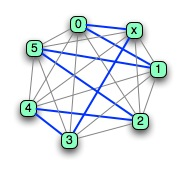# Graph Decomposition and Turning Cycles

One thing that we often want to do is break a graph into pieces in a way that preserves
the structural relations between the vertices in any part. Doing that is called
*decomposing* the graph. Decomposition is a useful technique because many ways
of studying the structure of a graph, and many algorithms over graphs can work by
decomposing the graph, studying the elements of the decomposition, and then combining
the results.
To be formal: a graph G can be decomposed into a set of subgraphs {G1, G2, G3, …}, where the edges of each of the Gis are
*disjoint* subsets of the edges of G. So in a decomposition of G, *vertices* can be shared between elements of the decomposition, but *edges* cannot.

So, for example, here is K5, and a decomposition of it into three subgraphs. To make it clearer, the original graph has its edges colored to match the colors of the vertices in the subgraph it belongs to in the decomposition.We can prove that any complete graph K2n+1 can be decomposed into n hamiltonian cycles. (As a reminder, a hamiltonion cycle is a path through a graph with the same first and last vertex, and which visits every other vertex in the graph exactly once.)The proof
is another turning proof. I’ll just show you the basic structure of the hamiltonian cycle that we’ll use, with K7 (2n+1 for n=3) as an example. You can see the cycle nice and clearly. If you turn the graph so that x is matched with 1, you get another cycle; if you turn again so that x is matched with 2, you’ll get a third cycle. If you turn again, you’ll get x matched with three – which is a repeat of the cycle we started with. In any complete graph with 2n+1 vertices, after n turns, you’ll get a repeat. Further, we know that the complete graph for K2n+1 has (2n+1) choose 2 – or n×(2n+1) edges. Each hamiltonion cycle has 2n+1 edges, so there *can’t* be more than N of them.
Using a simple variation of this, we can also show that any complete graph K2n
has N hamiltonian *paths*. (A hamiltonian path is a path that touches each vertex once, but it doesn’t have to be a cycle.) The way we can prove that is really clever. Take the turning proof for 2n+1 and the hamiltonian cycles – and *delete* node x. Then it will produce N hamiltonian paths on a complete graph with 2n vertices.

## 0 thoughts on “Graph Decomposition and Turning Cycles”

1.David

Regrading the number of edges in a complete graph – K2n+1 has (2n+1) choose 2. Shouln’t that be (2n+1) choose n. Nice example, thanks.

2.Morgan

(2n+1) choose 2, because each edge uses two of the 2n+1 vertices – unless I’m very much mistaken.

3.Xanthir, FCD

You are correct, Morgan. You’re trying to find every pair of vertices, so it’s choose 2.

4.David

Yep, thanks both. I didn’t read the ‘choose 2’ part of the sentence in the context of its combinatorial meaning, which is obvious now. Instead, I read it as – for each vertex pick two with which to connect to.